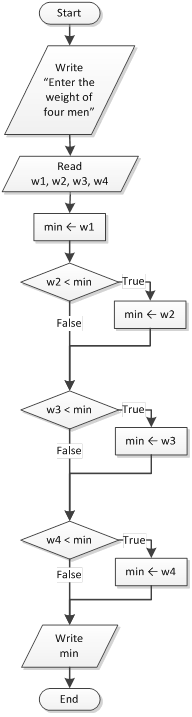Suppose there are some men and you want to find the lightest one. Let’s say that each one of them comes by and tells you his weight. What you must do is, memorize the weight of the first person that has come by and for each new person, you have to compare his weight with the one that you keep memorized. If he is heavier, you ignore his weight. However, if he is lighter, you need to forget the previous weight and memorize the new one. The same procedure continues until all the people have come by.

Let’s ask four men to come by at a random order. Assume that their weights, in order of appearance, are 165, 170, 160, and 180 pounds.

 Procedure Value of Variable `min` in Your Mind! The first person comes by. He weighs 165 pounds. Keep his weight in your mind (imagine a variable in your mind named min.) `min` = 165 The second person comes by. He weighs 170 pounds. He does not weigh less than the weight you are keeping in variable min, so you must ignore his weight. Variable min in your mind still contains the value 165. `min` = 165 The third person comes by. He weighs 160 pounds, which is less than the weight you are keeping in variable min, so you must forget the previous value and keep the value 160 in variable min. `min` = 160 The fourth person comes by. He weighs 180 pounds. He does not weigh less than the weight you are keeping in variable min, so you must ignore his weight. Variable min still contains the value 160. `min` = 160

When the procedure finishes, the variable `min` in your mind contains the weight of the lightest man!

Following are the flowchart and the corresponding program that prompts the user to enter the weight of four people and then finds and displays the lightest weight.## PHP

```<?php
echo "Enter the weight of four men:";

\$w1 = trim(fgets(STDIN));
\$w2 = trim(fgets(STDIN));
\$w3 = trim(fgets(STDIN));
\$w4 = trim(fgets(STDIN));

//memorize the weight of the first person
\$min = \$w1;

//if second one is lighter, forget
//everything and memorize this weight
if (\$w2 < \$min) {
\$min = \$w2;
}

//if third one is lighter, forget
//everything and memorize this weight
if (\$w3 < \$min) {
\$min = \$w3;
}

//if fourth one is lighter, forget
//everything and memorize this weight
if (\$w4 < \$min) {
\$min = \$w4;
}

echo \$min;

?>```

## Java

```public static void main(String[] args) throws java.io.IOException {
double w1, w2, w3, w4, min;

System.out.print("Enter the weight ");
System.out.println("of four men:");

//memorize the weight of the first person
min = w1;

//if second one is lighter, forget
//everything and memorize this weight
if (w2 < min) {
min = w2;
}

//if third one is lighter, forget
//everything and memorize this weight
if (w3 < min) {
min = w3;
}

//if fourth one is lighter, forget
//everything and memorize this weight
if (w4 < min) {
min = w4;
}

System.out.println(min);
}```

## C++

```#include <iostream>
using namespace std;
int main() {
double w1, w2, w3, w4, min;

cout << "Enter the weight ";
cout << "of four men:" << endl;

cin >> w1;
cin >> w2;
cin >> w3;
cin >> w4;

//memorize the weight of the first person
min = w1;

//if second one is lighter, forget
//everything and memorize this weight
if (w2 < min) {
min = w2;
}

//if third one is lighter, forget
//everything and memorize this weight
if (w3 < min) {
min = w3;
}

//if fourth one is lighter, forget
//everything and memorize this weight
if (w4 < min) {
min = w4;
}

cout << min;

return 0;
}```

## C#

```static void Main() {
double w1, w2, w3, w4, min;

Console.Write("Enter the weight ");
Console.WriteLine("of four men:");

//memorize the weight of the first person
min = w1;

//if second one is lighter, forget
//everything and memorize this weight
if (w2 < min) {
min = w2;
}

//if third one is lighter, forget
//everything and memorize this weight
if (w3 < min) {
min = w3;
}

//if fourth one is lighter, forget
//everything and memorize this weight
if (w4 < min) {
min = w4;
}

Console.Write(min);

}```

## Visual Basic

```Sub Main()
Dim w1, w2, w3, w4, min As Double

Console.Write("Enter the weight ")
Console.WriteLine("of four men:")

'memorize the weight of the first person
min = w1

'If second one is lighter, forget
'everything and memorize this weight
If w2 < min Then
min = w2
End If

'If third one is lighter, forget
'everything and memorize this weight
If w3 < min Then
min = w3
End If

'If fourth one is lighter, forget
'everything and memorize this weight
If w4 < min Then
min = w4
End If

Console.Write(min)

End Sub```

## Python

```print("Enter the weight of four men:")

w1 = float(input())
w2 = float(input())
w3 = float(input())
w4 = float(input())

#memorize the weight of the first person
minimum = w1

#If second one is lighter, forget
#everything and memorize this weight
if w2 < minimum:
minimum = w2

#If third one is lighter, forget
#everything and memorize this weight
if w3 < minimum:
minimum = w3

#If fourth one is lighter, forget
#everything and memorize this weight
if w4 < minimum:
minimum = w4

print(minimum)```

Notice: You can find the maximum instead of the minimum value by simply replacing the “less than” with a “greater than” operator in all Boolean expressions.